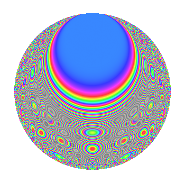# Properties

 Label 804.2.y.aLevel 804 Weight 2 Character orbit 804.y Analytic conductor 6.420 Analytic rank 0 Dimension 100 CM No

# Related objects

## Newspace parameters

 Level: $$N$$ = $$804 = 2^{2} \cdot 3 \cdot 67$$ Weight: $$k$$ = $$2$$ Character orbit: $$[\chi]$$ = 804.y (of order $$33$$ and degree $$20$$)

## Newform invariants

 Self dual: No Analytic conductor: $$6.41997232251$$ Analytic rank: $$0$$ Dimension: $$100$$ Relative dimension: $$5$$ over $$\Q(\zeta_{33})$$ Sato-Tate group: $\mathrm{SU}(2)[C_{33}]$

## $q$-expansion

The dimension is sufficiently large that we do not compute an algebraic $$q$$-expansion, but we have computed the trace expansion.

 $$\operatorname{Tr}(f)(q) =$$ $$100q - 10q^{3} + 2q^{5} - 3q^{7} - 10q^{9} + O(q^{10})$$ $$\operatorname{Tr}(f)(q) =$$ $$100q - 10q^{3} + 2q^{5} - 3q^{7} - 10q^{9} - 13q^{11} - 3q^{13} - 9q^{15} - 44q^{17} - 16q^{19} - 3q^{21} - 16q^{23} + 28q^{25} - 10q^{27} - 7q^{29} + 20q^{31} - 2q^{33} - 19q^{35} - 22q^{37} - 3q^{39} - 14q^{41} - 27q^{43} + 2q^{45} + 4q^{47} - 92q^{49} + 22q^{51} + 8q^{53} - 13q^{55} + 17q^{57} + 22q^{59} + 17q^{61} - 3q^{63} + 56q^{65} - 14q^{67} + 17q^{69} - q^{71} + 26q^{73} + 28q^{75} + 112q^{77} + 69q^{79} - 10q^{81} + 15q^{83} + 69q^{85} + 4q^{87} + 73q^{89} - 40q^{91} - 13q^{93} + 59q^{95} + 29q^{97} - 2q^{99} + O(q^{100})$$

## Embeddings

For each embedding $$\iota_m$$ of the coefficient field, the values $$\iota_m(a_n)$$ are shown below.

For more information on an embedded modular form you can click on its label.

Label $$a_{2}$$ $$a_{3}$$ $$a_{4}$$ $$a_{5}$$ $$a_{6}$$ $$a_{7}$$ $$a_{8}$$ $$a_{9}$$ $$a_{10}$$
49.1 0 0.841254 + 0.540641i 0 −0.185691 1.29151i 0 −3.04666 0.290921i 0 0.415415 + 0.909632i 0
49.2 0 0.841254 + 0.540641i 0 −0.144003 1.00156i 0 −0.962860 0.0919419i 0 0.415415 + 0.909632i 0
49.3 0 0.841254 + 0.540641i 0 −0.138288 0.961813i 0 4.23021 + 0.403936i 0 0.415415 + 0.909632i 0
49.4 0 0.841254 + 0.540641i 0 0.237705 + 1.65327i 0 −3.35218 0.320095i 0 0.415415 + 0.909632i 0
49.5 0 0.841254 + 0.540641i 0 0.513618 + 3.57229i 0 −0.127258 0.0121517i 0 0.415415 + 0.909632i 0
73.1 0 0.415415 0.909632i 0 −4.17942 1.22719i 0 1.04002 0.200448i 0 −0.654861 0.755750i 0
73.2 0 0.415415 0.909632i 0 −1.28965 0.378675i 0 −0.228496 + 0.0440390i 0 −0.654861 0.755750i 0
73.3 0 0.415415 0.909632i 0 −0.620947 0.182327i 0 −2.64850 + 0.510456i 0 −0.654861 0.755750i 0
73.4 0 0.415415 0.909632i 0 0.921315 + 0.270523i 0 1.11642 0.215173i 0 −0.654861 0.755750i 0
73.5 0 0.415415 0.909632i 0 3.28440 + 0.964386i 0 2.82322 0.544130i 0 −0.654861 0.755750i 0
121.1 0 −0.654861 + 0.755750i 0 −2.40665 + 1.54666i 0 1.93826 + 0.775962i 0 −0.142315 0.989821i 0
121.2 0 −0.654861 + 0.755750i 0 −1.55544 + 0.999620i 0 −0.0528822 0.0211708i 0 −0.142315 0.989821i 0
121.3 0 −0.654861 + 0.755750i 0 −0.236859 + 0.152220i 0 −1.28374 0.513932i 0 −0.142315 0.989821i 0
121.4 0 −0.654861 + 0.755750i 0 2.57269 1.65337i 0 −1.89045 0.756823i 0 −0.142315 0.989821i 0
121.5 0 −0.654861 + 0.755750i 0 3.18825 2.04896i 0 4.41412 + 1.76715i 0 −0.142315 0.989821i 0
157.1 0 −0.142315 + 0.989821i 0 −0.926989 2.02982i 0 2.57416 2.45446i 0 −0.959493 0.281733i 0
157.2 0 −0.142315 + 0.989821i 0 −0.793528 1.73758i 0 −0.366312 + 0.349278i 0 −0.959493 0.281733i 0
157.3 0 −0.142315 + 0.989821i 0 0.336386 + 0.736583i 0 −0.860971 + 0.820934i 0 −0.959493 0.281733i 0
157.4 0 −0.142315 + 0.989821i 0 0.716491 + 1.56890i 0 0.876788 0.836015i 0 −0.959493 0.281733i 0
157.5 0 −0.142315 + 0.989821i 0 1.26894 + 2.77859i 0 −3.37248 + 3.21566i 0 −0.959493 0.281733i 0
See all 100 embeddings
 $$n$$: e.g. 2-40 or 990-1000 Embeddings: e.g. 1-3 or 793.5 Significant digits: Format: Complex embeddings Normalized embeddings Satake parameters Satake angles

## Inner twists

This newform does not have CM; other inner twists have not been computed.

## Hecke kernels

This newform can be constructed as the kernel of the linear operator $$T_{5}^{100} - \cdots$$ acting on $$S_{2}^{\mathrm{new}}(804, [\chi])$$.